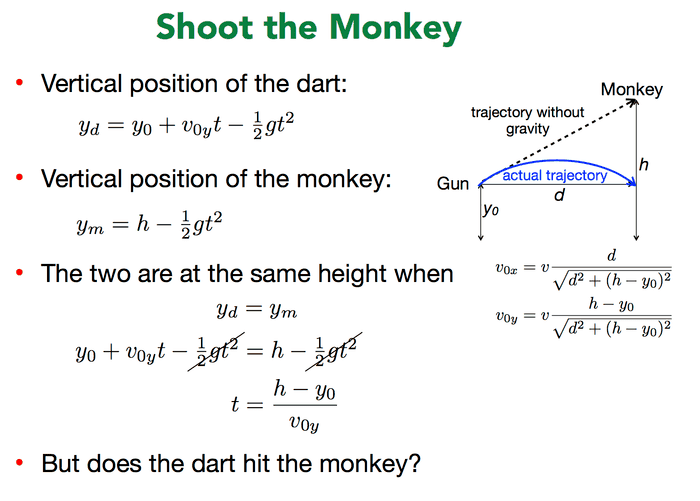# Trajectory of dartI don't quite get, where does the V0x=v * d/(sqrt(d^2+(h-y0)^2) come from?

also, what does (h-y0) equal to? t/v0y?

PeterDonis
Mentor
where does the V0x=v * d/(sqrt(d^2+(h-y0)^2) come from?

It's the Pythagorean theorem; the velocity ##v## is being split up into its horizontal and vertical components. The ratio of the components will be the same as the ratio of the horizontal and vertical lengths of the triangle in the diagram to the length of the hypotenuse.

•